# Pointers to Function and Function Arguments

So far we have discussed about pointers to variables and data objects. Pointers can also be used for referring functions. These types of pointers have wide range of use. Suppose we have to make changes to some array or variable those are used in the program without having any temporary variables, then the only option to do so is to pass those arguments as pointers. Hence function will get the address of the variable or array and it starts modifying data at that location without creating any copy of them. Hence those changes will automatically reflect in the calling program.

Simple pointer to a function can be illustrated by using an example of swap function. Suppose we have a swap function to swap two integers. In the below program we can see that a function fn_swap is written to swap two integer values. In the main function, a function pointer fn_swapPtr is declared and is pointing to the function fn_swap. Now we call this pointer function, then it will point to the function fn_swap and it will be called.

```#include <stdio.h>

void fn_swap (int *x, int *y) {// argument is a pointer
int intTemp;

intTemp = *x;
*x = *y;
*y = intTemp;
}

int main ()
{
int intX = 30;
int intY = 70;

void(*fn_swapPtr) (int *, int *) = fn_swap; // declare a function pointer to point to function fn_swap

printf("Variables BEFORE Swapping\n");
printf("--------------------------\n");
printf("intX = %d	", intX);
printf("intY = %d	", intY);

fn_swapPtr(&intX, &intY); // function pointer fn_swapPtr is called to call the function fn_swap

printf("\n\variables AFTER Swapping\n");
printf("--------------------------\n");
printf("intX = %d	", intX);
printf("intY = %d	", intY);

return 0;
}```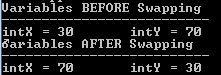Let us see more details about this function pointer. Simply calling a function just by declaring function pointer will not demonstrate its real use while programming. Above example shows how to declare and access a function pointer. One of the examples where real use of function pointer comes into picture is arithmetic operation where we have add, subtract, multiply and divide. Suppose a program is written to accept two numbers and operation to be performed from the user, and depending on the operation entered corresponding function is called. Typical program without any pointers is as shown below. Switch case statement is used to determine which function to be called.

```#include <stdio.h>

{
return (x + y);
}

int subtraction(int x, int y)
{
return (x / y);
}

int multiply(int x, int y)
{
return (x * y);
}

int divide(int x, int y)
{
return (x / y);
}

int main()
{
int intX, intY, intResult;
int intOption;

printf("Enter the two Numbers: ");
scanf("%d", &intX);
scanf("%d", &intY);

printf("0: Add \n 1: Subtract \n 2: Multiply \n 3: Divide\n");
printf("Enter the operation to be performed from above list:\n");
scanf("%d", &intOption);

switch (intOption)
{
case 0:  intResult = addition(intX, intY); break;
case 1:  intResult = subtraction(intX, intY); break;
case 2:  intResult = multiply(intX, intY); break;
case 3:  intResult = divide(intX, intY); break;
}

printf("Result = %d", intResult);
}```

Suppose we create a function pointer *operationPtr. Then above program can be changed as below:

```#include <stdio.h>

{
return (x + y);
}

int subtraction(int x, int y)
{
return (x / y);
}

int multiply(int x, int y)
{
return (x * y);
}

int divide(int x, int y)
{
return (x / y);
}

int main()
{
int intX, intY, intResult;
int intOption;

int(*operationPtr)(int x, int y) = { addition, subtraction, multiply, divide }; // declaring a array of function pointer

printf("Enter the two Numbers: ");
scanf("%d", &intX);
scanf("%d", &intY);

printf("0: Add \n 1: Subtract \n 2: Multiply \n 3: Divide\n");
printf("Enter the operation to be performed from above list:\n");
scanf("%d", &intOption);

intResult = operationPtr[intOption](intX, intY); // calls the respective function depending upon the option entered

printf("Result = %d", intResult);
}```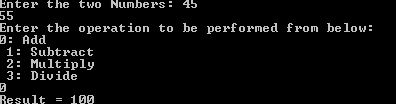We can see from above example that,  use of function pointer has simplified the program as well as it performs the selected operation too. This how we create a function pointer and use them in the program. We can notice here that the function pointer is used to implement different functions which are similar in their structure. In other words, function pointers are useful when a program has polymorphism. It makes the coding easy in those cases.

Now let us see how to pass function pointer as argument. Let us consider the same swap function as above. Now we have two swap functions – one to swap integers and one to swap characters. In both the functions, logic for swapping is the same. But the argument passed to the function is of different datatype. Hence we cannot use same swap function to swap the data, and we need two separate swap functions.

intTemp = intX;

intX = intY;

intY = intTemp;

Even though the logic is same the datatypes are different. But if we make the function independent of datatype, then our task of swapping becomes easier. We need not worry about the datatypes passed to function.  If we make these arguments as pointers, then they will point to the address locations rather than actual value and our task of swapping becomes easier – just interchange the addresses of variables rather than values. This looks simpler. But do you think it will get rid of datatypes passed to function? No, it does not eliminate datatype because we need to specify the datatype that the pointer is pointing to. Observe the snippets below to swap integers and characters by passing pointers. They have their datatypes specified in the argument.

```void fn_swap (int *x, int*y) {// argument is a pointer
int intTemp;

intTemp = *x;
*x = *y;
*y = intTemp;
}```

```void fn_swap (char *x, char *y) {// argument is a pointer
char charTemp;

charTemp = *x;
*x = *y;
*y = charTemp;
}```

Our goal here is get rid of datatypes in the function argument and make the function datatype free arguments. This will help to call the functions irrespective of the datatype from the main function. So we have to make these arguments as void pointer rather than integer or character pointers. But passing the argument as void will make the function independent of datatypes, but when we are using those arguments in the function, we should know the datatypes. We cannot interchange them without knowing what kind of data value it contains. Hence we need to cast the arguments to respective datatypes inside the function. Hence the above swap function will change as below.

```void fn_intswap (void *x, void *y) {// argument is a pointer
int intTemp;
int *x1 = (int *)x;
int *y1 = (int *)y;

intTemp = *x1;
*x1 = *y1;
*y1 = intTemp;
}```
```void fn_charswap(void *x, void *y) {// argument is a pointer
char charTemp;
char *x1 = (char *)x;
char *y1 = (char *)y;

charTemp = *x1;
*x1 = *y1;
*y1 = charTemp;
}```

Now both the function arguments are datatype free and we need not worry about the datatype passed to it. Now how to call these functions and pass the arguments which are void? We will create a function pointer which will point to either of these functions depending on the arguments passed. i.e.; we will create a function pointer *fnPtr, which will accept void pointer arguments and will made to point to either fn_intswap or fn_charswap at run time.

`void(*fnPtr)(void *,  void *)`

We can see here that its arguments are also void. How the compiler will know which datatypes are passed to it? Compiler itself cannot know which function to be called. The user / developer has to code it in such a way that compiler should know which function to be called. One method of coding this is using same method that we used in arithmetic operation. Let us see here another method by passing function pointer itself as an argument. So, we will write another function fn_swap, which will accept the variables to be swapped (independent of datatypes – void), and the function to be called by passing the pointer to that function. This is a simple function which is calling the function that the function pointer is pointing to. i.e.; if the function pointer is pointing to fn_intswap then that function will be called, if it is pointing to fn_charswap then that function will be called.

```void fn_swap(void *x, void *y, void(*fnPtr)(void *,  void *)){
fnPtr(x, y); // Calls the respective function
}```

How we will make the function pointer to point to respective functions? This is done in the main program while placing the function call. Here fn_swap passes the pointer to the respective function as argument.

```fn_swap(&intX, &intY, fn_intswap);// swap integers using function pointer
fn_swap(&charX, &charY, fn_charswap); // swap integers using function pointer```

```#include <stdio.h>

void fn_charswap(void *x,  void *y) {// argument is a pointer
char charTemp;
char *x1 = (char *)x;
char *y1 = (char *)y;

charTemp = *x1;
*x1 = *y1;
*y1 = charTemp;
}

void fn_intswap(void *x,  void *y) {// argument is a pointer
int intTemp;
int *x1 = (int *)x;
int *y1 = (int *)y;

intTemp = *x1;
*x1 = *y1;
*y1 = intTemp;
}

void fn_swap(void *x, void *y, void(*fnPtr)(void *,  void *)){// passing function pointer as argument
fnPtr(x, y);
}

int main()
{
char charX = 'C';
char charY = 'P';
int intX = 12;
int intY = 67;

printf("Variables BEFORE Swapping\n");
printf("--------------------------\n");
printf("charX = %c	", charX);
printf("charY = %c	", charY);
printf("\nintX = %d	", intX);
printf("intY = %d	", intY);

fn_swap(&intX, &intY, fn_intswap);// swap integers using integer function pointer
fn_swap(&charX, &charY, fn_charswap); // swap integers using character function pointer
//fn_swap(&intX, &intY, fn_charswap);

printf("\n\nVariables AFTER Swapping\n");
printf("--------------------------\n");
printf("charX = %c	", charX);
printf("charY = %c	", charY);
printf("\nintX = %d	", intX);
printf("intY = %d	", intY);

return 0;
}```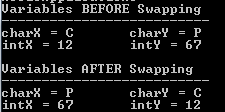Calling the integer function or character function in the main program is straight forward. But what will be the output of calling the function fn_swap with argument as shown below ?

`fn_swap(&intX, &intY, fn_charswap);`

Here variables passed to the function are integers but function pointer points to character function. Will this swap integer values? Modify the code as below in the main program above and execute the code. What is the result? Did it call fn_charswap? Did it swap the integer value or character value or no change ?

```//fn_swap(&intX, &intY, fn_intswap);// swap integers using function pointer
fn_swap(&charX, &charY, fn_charswap); // swap integers using function pointer
fn_swap(&intX, &intY, fn_charswap);```

It has swapped the integer values! But we had passed function pointer to character swap. Still it has swapped integer values. How did this happen? This is because of independency of datatype and pointers to the variable. That means, we have not specified the datatype of variables while passing to the function and it is considered as void. In addition pointers to those variables are passed which means address of those variables is passed to the function- address of intX and intY. Hence when main program calls the function fn_swap, addresses of these two variables are passed. Even though function pointer passed to fn_swap is fn_charswap, it does not get the actual value of the variables. It sees only their memory address and swaps the addresses. But uses casting inside it because, it cannot simply interchange the address for void variables.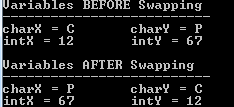From the above example, we understand that we do not need two functions to swap integer and characters. We can use only one common function to swap any type of variables by passing the pointers to the variable. Hence we can modify above code to have only one swap function.

P.S: fn_swap is retained to show how to pass function pointer as argument. But this function can also be eliminated (as shown below) to make the code simpler. Rest of the code remains the same as in below box.

```#include <stdio.h>

void fn_swapVariables(void *x, void *y) {// argument is a pointer
int intTemp;
int *x1 = (int *)x; // could be casted to any datatype, this actually does not matter as address of variables are considered here.
int *y1 = (int *)y;

intTemp = *x1;
*x1 = *y1;
*y1 = intTemp;
}

void fn_swap(void *x, void *y, void(*fnPtr)(void *, void *)){// passing function pointer as argument
fnPtr(x, y);
}

int main()
{
char charX = 'C';
char charY = 'P';
int intX = 12;
int intY = 67;

printf("Variables BEFORE Swapping\n");
printf("--------------------------\n");
printf("charX = %c	", charX);
printf("charY = %c	", charY);
printf("\nintX = %d	", intX);
printf("intY = %d	", intY);

fn_swap(&intX, &intY, fn_swapVariables);// swap integers using integer function pointer
fn_swap(&charX, &charY, fn_swapVariables); // swap integers using character function pointer

// Above 2 lines of function call can also be called as below
//fn_swapVariables(&intX, &intY);// swap integers without passing function pointer
//fn_swapVariables(&charX, &charY);

printf("\n\nVariables AFTER Swapping\n");
printf("--------------------------\n");
printf("charX = %c	", charX);
printf("charY = %c	", charY);
printf("\nintX = %d	", intX);
printf("intY = %d	", intY);

return 0;
}```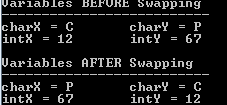We can illustrate function pointer as an argument for arithmetic operation as below.

```#include <stdio.h>

{
return (x + y);
}

int subtraction(int x, int y)
{
return (x / y);
}

int multiply(int x, int y)
{
return (x * y);
}

int divide(int x, int y)
{
return (x / y);
}

int fnOperation(int x, int y, int(*fnPtr)(int, int)){ // function pointer is passed to the function
return (*fnPtr)(x, y);
}

int main()
{
int intX, intY, intResult;
int intOption;

printf("Enter the two Numbers: ");
scanf("%d", &intX);
scanf("%d", &intY);

printf("0: Add \n 1: Subtract \n 2: Multiply \n 3: Divide\n");
printf("Enter the operation to be performed from above list:\n");
scanf("%d", &intOption);

switch (intOption)
{ // call respective functions by passing the pointers to the function
case 0:  intResult = fnOperation(intX, intY, addition); break;
case 1:  intResult = fnOperation(intX, intY, subtraction); break;
case 2:  intResult = fnOperation(intX, intY, multiply); break;
case 3:  intResult = fnOperation(intX, intY, divide); break;
}

printf("Result = %d", intResult);
}```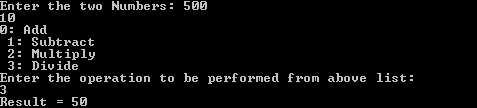Above example is different from swapping example. Here different arithmetic functions are used to perform the operation, and the function pointer is referred to respective function at run time depending on the option that the user enters. Hence we cannot eliminate any of the functions like we did it in swap, but it  gives the flexibility of calling the required function depending on user input. Here variables are passed by value rather than passing it as address.

Here developer need not know the type of variables, number of variables, and method of passing it to different arithmetic function. If he knows only fnOperation function, it is sufficient to perform all the operations. Also while calling fnOperation, the function pointers to other function does not know anything about the values passed  to it. It actually gets the value inside the function fnOperation when it is calling actual function at run time.

Translate »